# Whole PI comes from the Pi Unit Simulacrum

The Pi Unit Simulacrum makes you examine the nature of the unit, and leads directly to The Pi Paradox.

## Whole PI leads to a new understanding of Dimensions

Whole PI came from operating pi, π, in The Unit Simulacrum, to give the Pi Unit Simulacrum. The Unit Simulacrum makes you consider the nature of the unit in any Unit Definition. The Pi Unit Simulacrum makes you aware that there are two different definitions for the single symbol, π, leading to The Pi Paradox.

### The Pi Unit Simulacrum

Operating pi, π, in The Unit Simulacrum gives
The Pi Unit Simulacrum:

Simulacrum Sum of 1 and π = SimSum(1, π) = Sum ( 1 + π ) =
{Possibilities to Observe: 1, π, (1/π), (π/1);
Case 1: π <= 1, 1 >= π, (π/1) <= 1, (1/π) >= 1;
π ( 1 + 1/(π/1) ) or 1 ( 1 + (π/1) )
π ( 1 + (1/π) ) or 1 ( 1 + 1/(1/π) )
Case 2: π >= 1, 1 <= π, (π/1) >= 1, (1/π) <= 1;
π ( 1 + 1/(π/1) ) or 1 ( 1 + (π/1) )
π ( 1 + (1/π) ) or 1 ( 1 + 1/(1/π) ) ) }

Interpretation Matrix: Definitions of 1 and π, and relationships between them. 1 can be defined as the unit that defines π, making 1+ π The Unit Definition of π, also known as The Unit Simulacrum of π.
In our universally agreed to symbology, π is defined as the ratio of the circumference of a circle to its diameter. And π has an agreed upon value of 3.14159265...

Since π = 3.14159265..., it is always greater than 1.
π >= 1, so π is always Case 2.
When deciding which of the four (4) available Case 2 solutions to select, we look for the easiest answers. We first focus on the two solutions that have a "1" outside the parentheses. We can simply cancel out the 1's, thanks to the identity property of multiplication. The parentheses can then be removed.
That leaves the two solutions:
1 + (π/1) or 1 + 1/(1/π)

The two ratios in the Possibilities to Observe (Poss2Obs), (π/1) and (1/π),
were inverses and give inverse solutions.(π/1) and (1/π) both include a unit, 1, and π, so they invite the question: "What is the unit?"

Many people, even mathematicians, never stop to ponder that there are two different unit definitions for π. One is based on radius, r, and the other is based on diameter, d.
The fact that multiples of π can be in two different units leads to The Pi Paradox.

Using the Unit Circle circumference as an example, the circumference, C, has two definitions:
C=2πr1   and   C=πd1

The two unit circles have the same circumference, but give C = 2π·1 = π·1, and when the 1's cancel out, you see C = 2π =π.C=2π and C=π,
so
2π = π, or
6.2831... = 3.1415...
How can 2π = π ?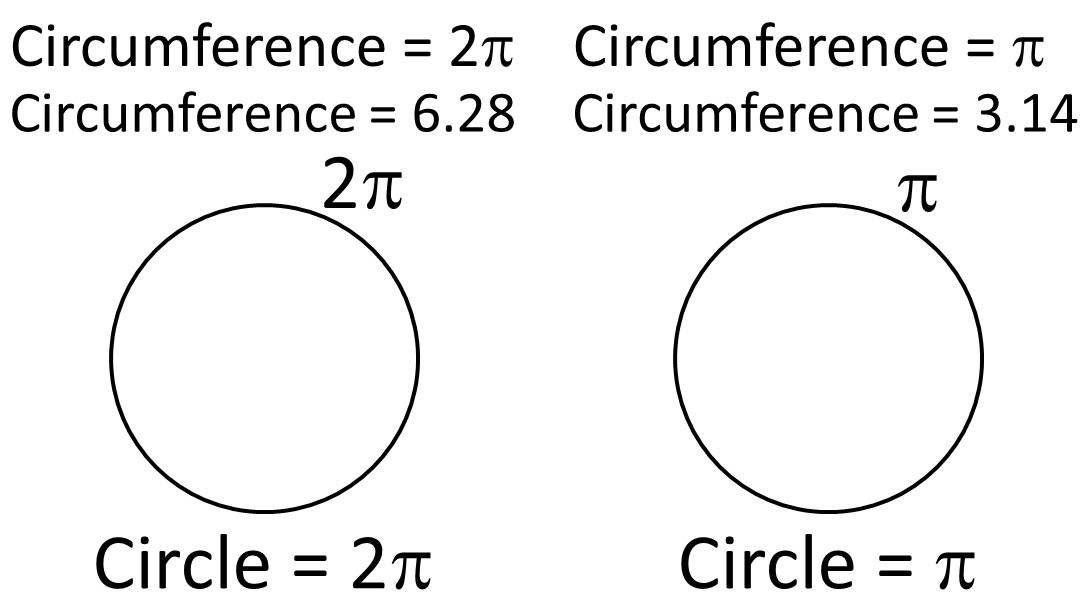Unless you explicitly state which unit it is based on, 2π could be one circle based on a unit r, or two circles based on a unit d. We have two definitions for the same unit symbol, π, leading to the paradox that 2π = π,### Whole PI solves The Pi Paradox

Since The Pi Paradox results from one symbol having two meanings, the solution is straightforward. Two meanings need two symbols, so I looked around for the perfect symbol, but never found it.

π is the symbol for the unit circle based on a unit radius (1). The unit circle based on diameter needs its own symbol. Since the second unit circle is based on the whole diameter, and not the radius, which is the First Deconstruction r = d x 1/(1+1), the second symbol for pi is called Whole PI.

# You've Been Living In The Deconstruction!!

Radius π is the Deconstruction, 1/(1+1) ! Whole PI is the Unification, 1 = 1/1 !

Since Whole PI is based on the whole unit circle, the whole unit circle is included in the symbol for Whole PI. Since there are three dimensions in 3D space, the symbol for Whole PI includes three diameters representing the three dimensions. PI is capitalized to differentiate it from pi and Pi, which can both apply to π.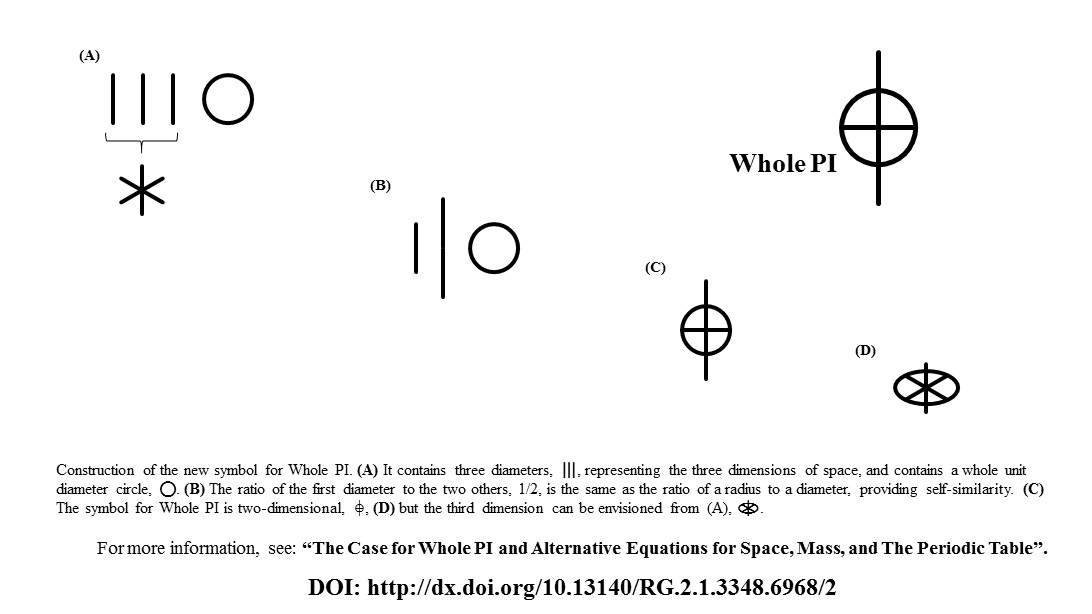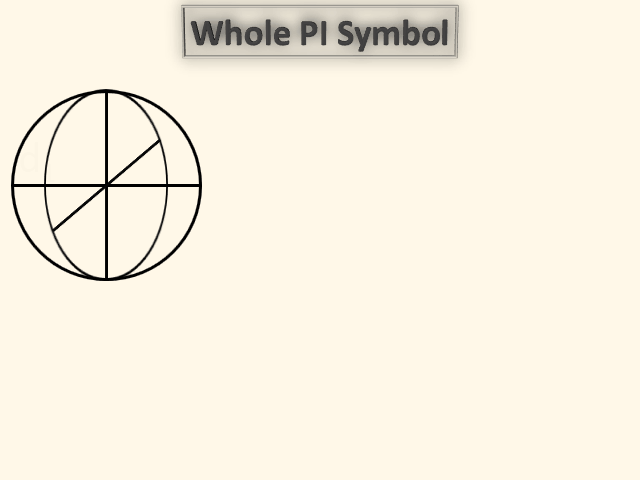### Whole PI leads to a new understanding of Dimensions

Ready to dive down the rabbit hole?### Here Is The Unit Circle We Learned in Elementary School: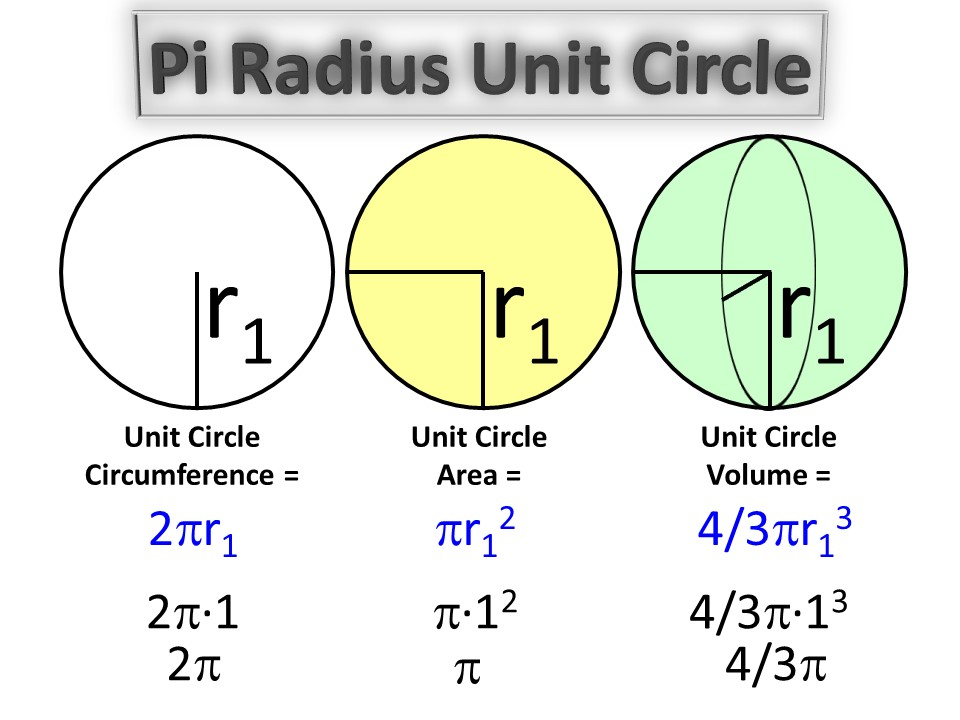Did you ever ask yourself why 4/3π for the 3rd dimension? Where did 4/3 come from? 4/3? Why? What is the pattern behind these dimension equations?
If you learn integral calculus, you can see how the three represents the post-integral dimension number and the four is a doubling, due to symmetry, of the pre-integral dimension.
But There's No Obvious Pattern!

When you think in terms of whole diameters and  Whole PI, you arrive at new equations that soon reveal a common pattern behind all equations.

When you put the dimensions in terms of diameter, you obtain the following equations:First, it is pretty easy to imagine the common pattern between the 2nd and 3rd dimensions. You can see that the bottom of the ratio is two times the power. Both the 2nd and 3rd dimension equations can easily be seen to be πdp/2p.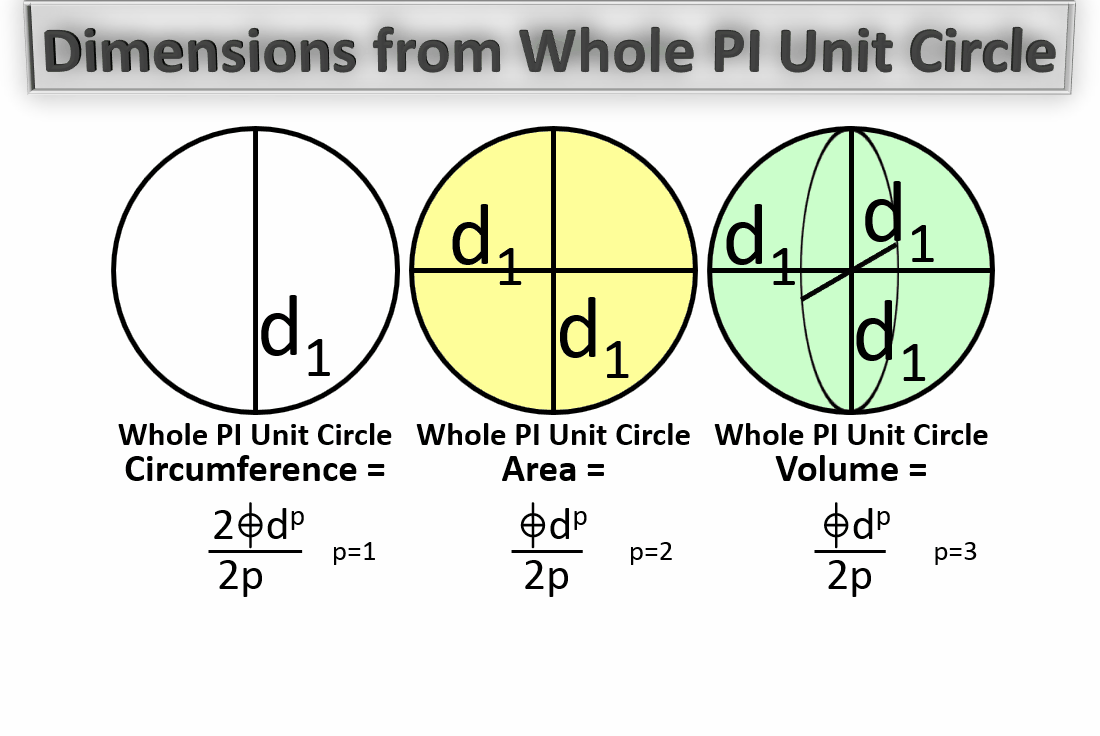To be continued.To be continued.

To be continued.

Once You've Seen the World through Whole PI, you may never see it the same again.

To be continued.

To be continued.